In :
import numpy as np
import pandas as pd

## Plotly plotting support
import plotly.plotly as py

# import plotly.offline as py
# py.init_notebook_mode()

import plotly.graph_objs as go
import plotly.figure_factory as ff

# Make the notebook deterministic
np.random.seed(42)


Notebook created by Joseph E. Gonzalez for DS100.

# Feature Engineering¶

In the next few notebooks we will explore a key part of data science, feature engineering: the process of transforming the representation of model inputs to enable better model approximation. Feature engineering enables you to:

1. encode non-numeric features to be used as inputs to common numeric models
2. capture domain knowledge (e.g., the perceived loudness or sound is the log of the intensity)
3. transform complex relationships into simple linear relationships

## Mapping from Domain to Range¶

In the supervised learning setting were are given $(X,Y)$ paris with the goal of learning the mapping from $X$ to $Y$. For example, given pairs of square footage and price we want to learn a function that captures (or at least approximates) the relationship between square feet and price. Our functional approximation is some form of typically parametric mapping from some domain to some range: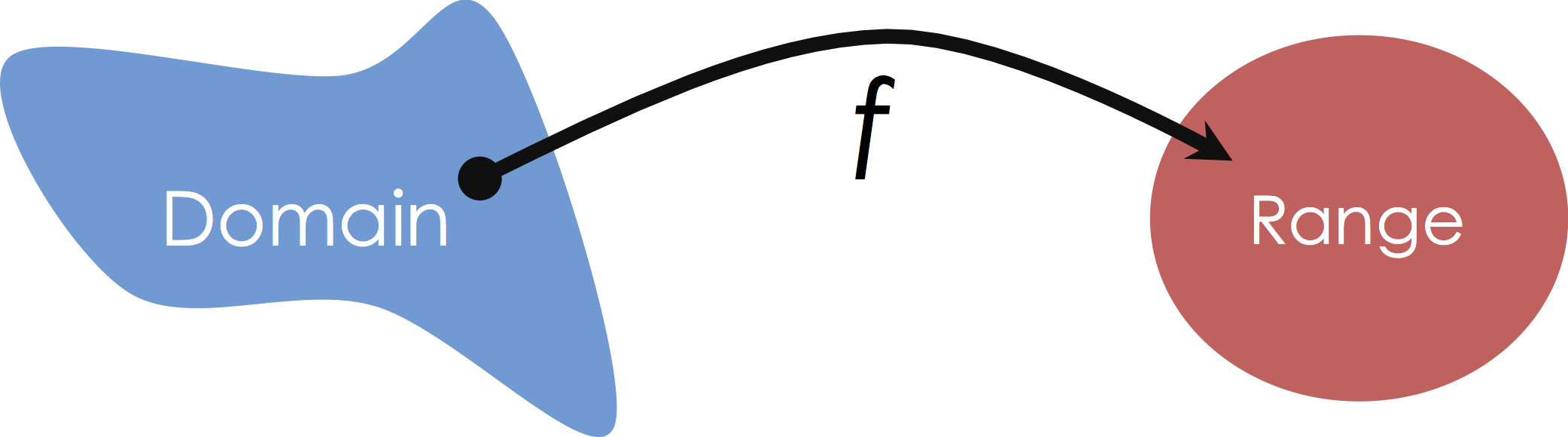In this class we will focus on Multiple Regression in which we consider mappings from potentially high-dimensional input spaces onto the real line (i.e., $y \in \mathbb{R}$):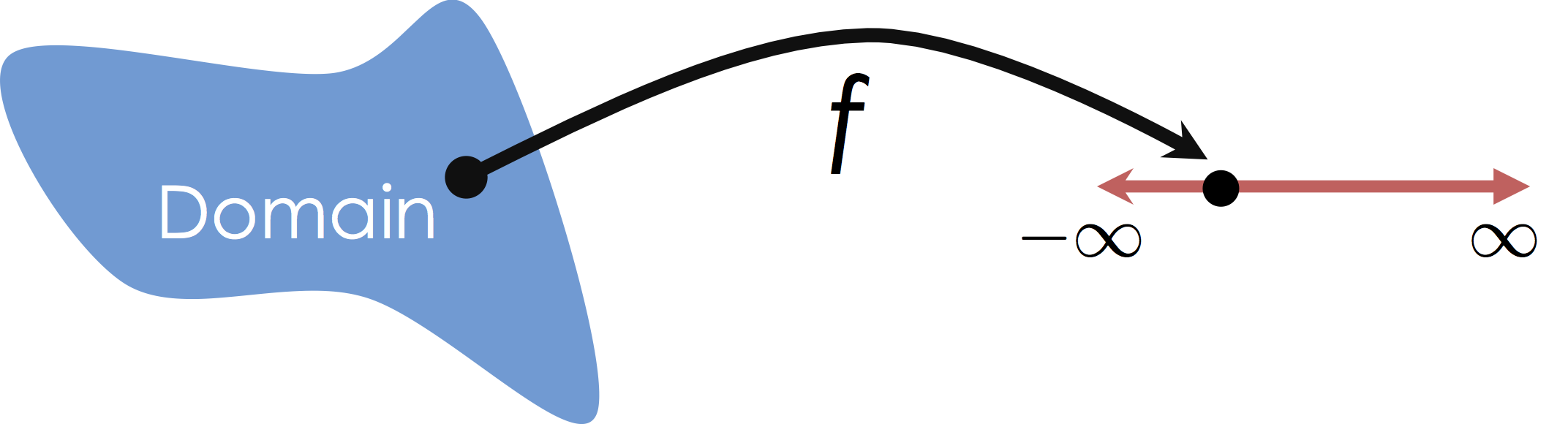It is worth noting that this is distinct from Multivariate Regression in which we are predicting multiple (confusing?) response values (e.g., $y \in \mathbb{R}^q$).

# What is the Domain (Features)¶

Suppose we are given the following table: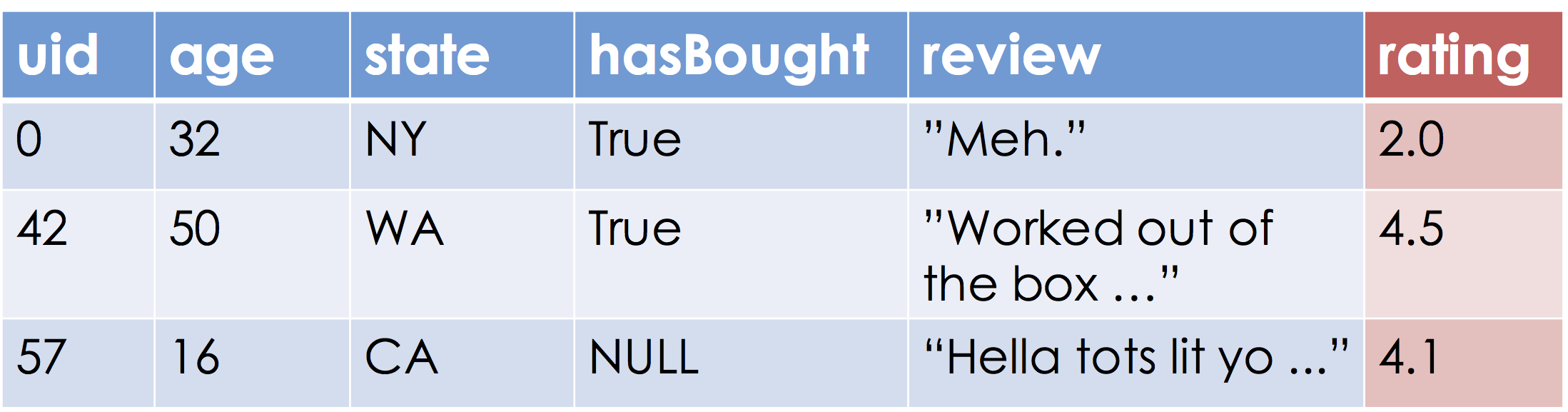Our goal is to learn a function that approximates the relationship between the blue and red columns. Let's assume the range, "Ratings", are the real numbers (this may be a problem if ratings are between [0, 5] but more on that later).

What is the domain of this function?

The schema of the relational model provides one possible answer:

RatingsData(uid INTEGER, age FLOAT,
state STRING, hasBought BOOLEAN,
review STRING, rating FLOAT)


Which would suggest that the domain is then:

$$\textbf{Domain} = \mathbb{Z} \times \mathbb{R} \times \mathbb{S} \times \mathbb{B} \times \mathbb{S} \times \mathbb{R}$$

Unfortunately, the techniques we have discussed so far and most of the techniques in machine learning and statistics operate on real-valued vector inputs $x \in \mathbb{R}^d$ (or for the statisticians $x \in \mathbb{R}^p$).

### Goal:¶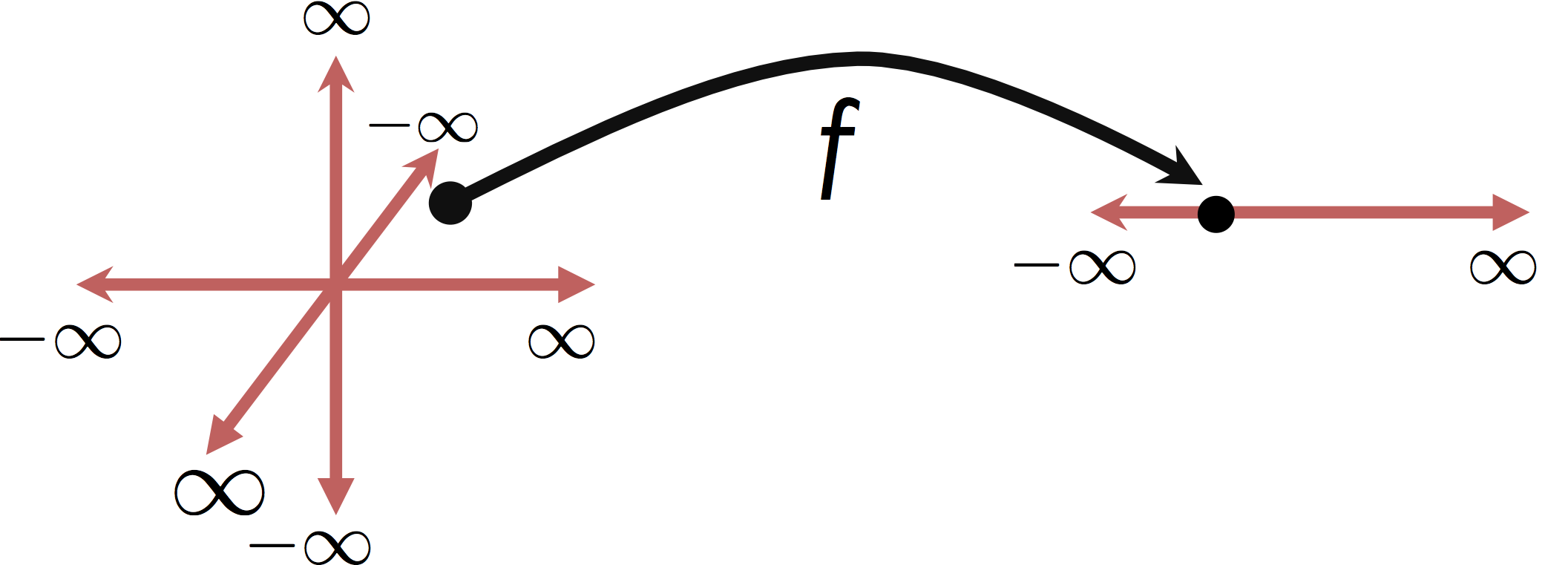Moreover, many of these techniques, especially the linear models we have been studying, assume the inputs are continuous variables in which the relative magnitude of the feature encode information about the response variable.

In the following we define several basic transformations to encode features as real numbers.

# Basic Feature Engineering: Get $\mathbb{R}$¶

Our first step as feature engineers is to translate our data into a form that encodes each feature as a continuous variable.

## The Uninformative Feature: uid¶

The uid was likely used to join the user information (e.g., age, and state) with some Reviews table. The uid presents several questions:

• What is the meaning of the uid number?
• Does the magnitude of the uid reveal information about the rating?

There are several answers:

1. Although numbers, identifiers are typically categorical (like strings) and as a consequence the magnitude has little meaning. In these settings we would either drop or one-hot encode the uid. We will return to feature dropping and one-hot-encoding in a moment.

2. There are scenarios where the magnitude of the numerical uid value contains important information. When user ids are created in consecutive order, larger user ids would imply more recent users. In these cases we might to interpret the uid feature as a real number.

## Dropping Features¶

While uncommon there are certain scenarios where manually dropping features might be helpful:

1. when the features does not to contain information associated with the prediction task. Dropping uninformative features can help to address over-fitting, an issue we will discuss in great detail soon.

2. when the feature is not available when at prediction time. For example, the feature might contain information collected after the user entered a rating. This is a common scenario in time-series analysis.

However in the absence of substantial domain knowledge, we would prefer to use algorithmic techniques to help eliminate features. We will discuss this more when we return to regularization.

## The Continuousage Feature¶

The age feature encodes the users age. This is already a continuous real number so no additional feature transformations are required. However, as we will soon see, we may introduce additional related features (e.g., indicators for various age groups or non-linear transformations).

## The Categoricalstate Feature¶

The state feature is a string encoding the category (one of the 50 states). How do we meaningfully encode such a feature as one or more real-numbers?

We could enumerate the states in alphabetical order AL=0, AK=2, ... WY=49. This is a form of dictionary encoding which maps each category to an integer. However, this would likely be a poor feature encoding since the magnitude provides little information about the rating.

Alternatively, we might enumerate the states based on their geographic region (e.g., lower numbers for coastal states.). While this alternative dictionary encoding may provide information there is better way to encode categorical features for machine learning algorithms.

# One-Hot Encoding¶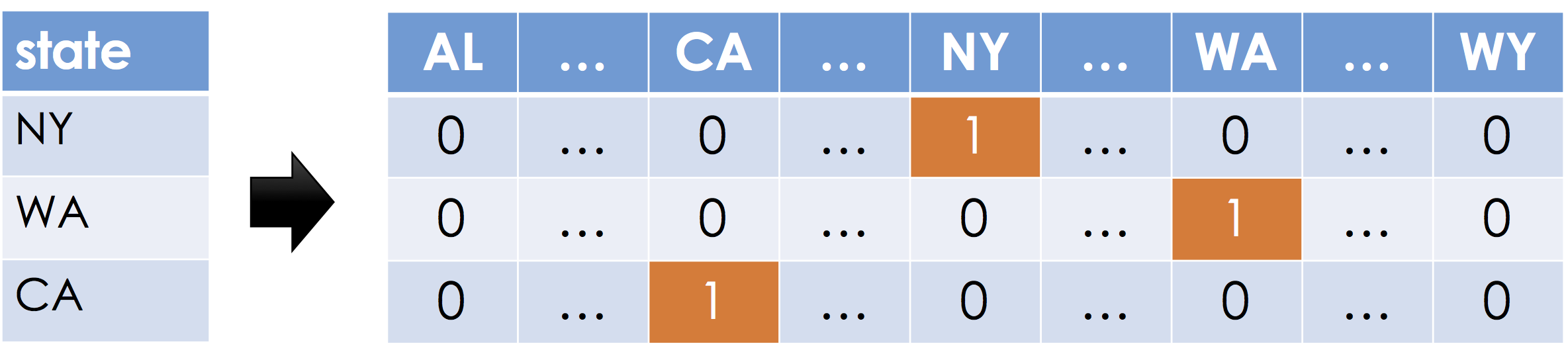One-Hot encoding, sometimes also called dummy encoding is a simple mechanism to encode categorical data as real numbers such that the magnitude of each dimension is meaningful. Suppose a feature can take on $k$ distinct values (e.g., $k=50$ for 50 states in the United Stated). For each distinct possible value a new feature (dimension) is created. For each record, all the new features are set to zero except the one corresponding to the value in the original feature.

The term one-hot encoding comes from a digital circuit encoding of a categorical state as particular "hot" wire: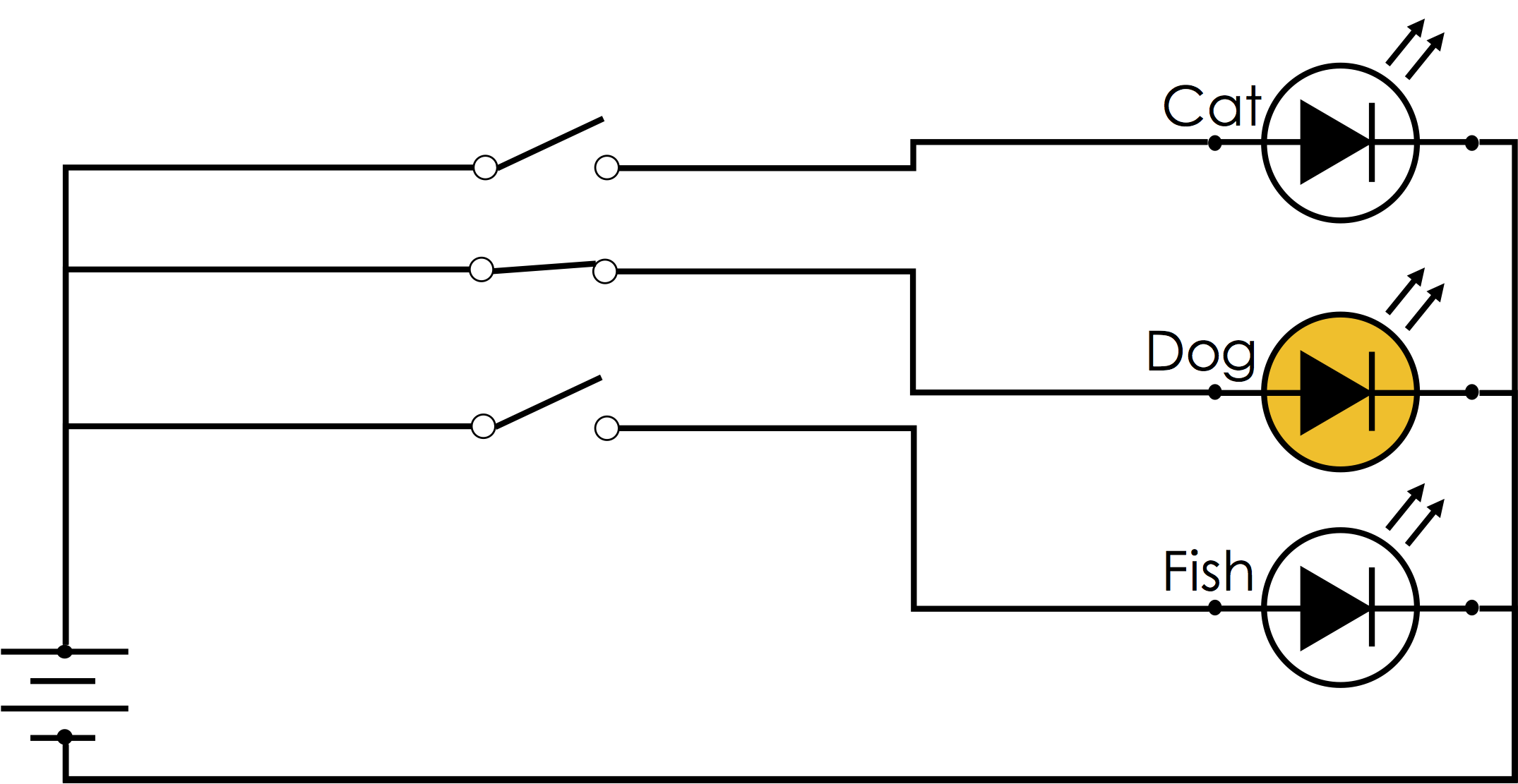The following is a relatively inefficient implementation:

In :
def one_hot_encoding(x, categories):
dictionary = dict(zip(categories, range(len(categories))))
enc = np.zeros(len(categories))
enc[dictionary[x]] = 1.0
return enc

categories = ["cat", "dog", "apple"]
one_hot_encoding("dog", categories)

Out:
array([ 0.,  1.,  0.])

Why is this inefficient? Think about a large number of states.

Answer: Here we are using a dense representation which does not make efficient use of memory

## One-Hot Encoding in Pandas¶

Here we create a toy dataframe of pets including their name and kind:

In :
df = pd.DataFrame({
"name": ["Goldy", "Scooby", "Brian", "Francine", "Goldy"],
"kind": ["Fish", "Dog", "Dog", "Cat", "Dog"],
"age": [0.5, 7., 3., 10., 1.]
}, columns = ["name", "kind", "age"])
df

Out:
name kind age
0 Goldy Fish 0.5
1 Scooby Dog 7.0
2 Brian Dog 3.0
3 Francine Cat 10.0
4 Goldy Dog 1.0

Pandas has a built in function to construct one-hot encodings called get_dummies

In :
pd.get_dummies(df['kind'])

Out:
Cat Dog Fish
0 0 0 1
1 0 1 0
2 0 1 0
3 1 0 0
4 0 1 0
In :
pd.get_dummies(df)

Out:
age name_Brian name_Francine name_Goldy name_Scooby kind_Cat kind_Dog kind_Fish
0 0.5 0 0 1 0 0 0 1
1 7.0 0 0 0 1 0 1 0
2 3.0 1 0 0 0 0 1 0
3 10.0 0 1 0 0 1 0 0
4 1.0 0 0 1 0 0 1 0

Issue: While the Pandas pandas.get_dummies function is very convenient and even retains meaningful column labels it has one key downside.

The get_dummies function does not take the dictionary of possible values and so will not produce the same encoding if applied across multiple dataframes with different values. This can be a big issue when rendering predictions on a new dataset.

## One-Hot Encoding in Scikit-Learn¶

Scikit-learn is a widely used machine learning package in Python and provides several implementations of feature encoders for categorical data.

### DictVectorizer¶

The DictVectorizer encodes dictionaries by taking keys that map to strings and applying a one-hot encoding.

In :
from sklearn.feature_extraction import DictVectorizer

vec_enc = DictVectorizer()
vec_enc.fit(df.to_dict(orient='records'))

Out:
DictVectorizer(dtype=<class 'numpy.float64'>, separator='=', sort=True,
sparse=True)
In :
vec_enc.transform(df.to_dict(orient='records')).toarray()

Out:
array([[  0.5,   0. ,   0. ,   1. ,   0. ,   0. ,   1. ,   0. ],
[  7. ,   0. ,   1. ,   0. ,   0. ,   0. ,   0. ,   1. ],
[  3. ,   0. ,   1. ,   0. ,   1. ,   0. ,   0. ,   0. ],
[ 10. ,   1. ,   0. ,   0. ,   0. ,   1. ,   0. ,   0. ],
[  1. ,   0. ,   1. ,   0. ,   0. ,   0. ,   1. ,   0. ]])
In :
vec_enc.get_feature_names()

Out:
['age',
'kind=Cat',
'kind=Dog',
'kind=Fish',
'name=Brian',
'name=Francine',
'name=Goldy',
'name=Scooby']

We can apply the dictionary vectorizer to new data:

In :
vec_enc.transform([
{"kind": "Cat", "name": "Goldy", "age": 35},
{"kind": "Bird", "name": "Fluffy"},
{"breed": "Chihuahua", "name": "Goldy"},
]).toarray()

Out:
array([[ 35.,   1.,   0.,   0.,   0.,   0.,   1.,   0.],
[  0.,   0.,   0.,   0.,   0.,   0.,   0.,   0.],
[  0.,   0.,   0.,   0.,   0.,   0.,   1.,   0.]])

Notice that the second record {"kind": "Bird", "name": "Fluffy"} has invalid categories and missing fields and it's encoding is entirely zero. Is this reasonable?

### Bonus: sklearn OneHotEncoder¶

The basic sklearn OneHotEncoder encodes a column of integers corresponding to category values. Therefore, we first need to dictionary encode the string values.

In :
# Convert the "kind" column into a category column
kind_codes = (
df['kind'].astype("category", categories=["Cat", "Dog","Fish"])
.cat.codes # Extract the category codes
)
kind_codes

Out:
0    2
1    1
2    1
3    0
4    1
dtype: int8
In :
from sklearn.preprocessing import OneHotEncoder

# Build an instance of the encoder
onehot = OneHotEncoder()

# Construct an integer column vector from the 'kind_codes' column
column_vec_kinds = np.array([kind_codes.values]).T

# Fit the encoder (which can be resued to transform other data)
onehot.fit(column_vec_kinds)

# Transform the column vector
onehot.transform(column_vec_kinds).toarray()

Out:
array([[ 0.,  0.,  1.],
[ 0.,  1.,  0.],
[ 0.,  1.,  0.],
[ 1.,  0.,  0.],
[ 0.,  1.,  0.]])

# One-Hot Encoding Icecream¶

Suppose you obtain the log of icecream sales for a popular icecream shop.The data consists of the flavor and topping, the total icecream mass (mass), and the price charged.

In :
icecream = pd.read_csv("icecream_train.csv")

Out:
flavor topping mass price
0 Chocolate Chocolate 2.5 2.50
1 Vanilla Chocolate 4.8 4.10
2 Strawberry Sprinkles 3.9 2.26
3 Strawberry Sprinkles 3.4 2.00
4 Chocolate Chocolate 1.6 1.80

### Predicting the price of icecream¶

How would you predict the price of icecream given the flavor, topping, and mass?

Let's start simple and focus on predicting the price from the mass:

In :
from sklearn import linear_model

# Train a linear regression modle to predict price from mass
reg_mass = linear_model.LinearRegression()
reg_mass.fit(icecream[['mass']], icecream['price'])

# Make predictions for each of the purchases in our dataset
yhat_mass = reg_mass.predict(icecream[['mass']])


### Analyze the fit¶

This is a fairly simple one-dimensional problem so we can plot the data.

In :
def plot_fit_line(x, y, model, filename):
# Data points
points = go.Scatter(name = "Data", x=x,y=y, mode='markers')
# Predictions for line
x_query = np.linspace(np.min(x), np.max(x), 1000)
y_query = model.predict(np.array([x_query]).T)
model_line = go.Scatter(name="Model", x=x_query, y=y_query)
# Residual line segments
residual_lines = [
go.Scatter(x=[x,x], y=[y,yhat],
mode='lines', showlegend=False,
line=dict(color='black', width = 0.5))
for (x, y, yhat) in zip(x, y, model.predict(np.array([x]).T))
]
return py.iplot([points, model_line] + residual_lines, filename=filename)

plot_fit_line(icecream['mass'], icecream['price'], reg_mass, "FE_Part1_0")

Out:
In :
residual = yhat_mass - icecream['price']
py.iplot(ff.create_distplot([residual], group_labels=['Residuals'], bin_size=0.1), filename="FE_Part1_1")

Out:

# RMSE and MAD¶

When plotting the prediction error it is common to compute the root mean squared error (RMSE) which is the square-root of the average squared loss over the training data.

$$\large \textbf{RMSE} = \sqrt{\frac{1}{n} \sum_{i=1}^n \left(Y_i - f_\theta(X_i)\right)^2}$$

The RMSE error in the units of $Y$ (in this case price) and is biased towards points with the highest error.

Another error metric that is a bit more robust is the median absolute devaiation (MAD) error.

$$\large \textbf{MAD} = \textbf{median}\left(Y_i - f_\theta(X_i)\right)$$

The RMSE error metric is closer to our squared loss objective and the MAD error is closer to an L1 loss and the corresponding Least Absolute Deviation Regression which we have not yet covered.

Let's take a look at both:

In :
def rmse(y, yhat):
return np.sqrt(np.mean((yhat-y)**2))

return np.median(np.abs(yhat - y))

In :
print("RMSE:", rmse(icecream['price'], yhat_mass))

RMSE: 0.537536988756


### Is this a good fit?¶

Often a very basic model is enough. However we notice something intresting.

At the same mass value there appears to be multiple icecream prices.

Why?

## Stratified Analysis¶

Given we have categorical data one thing we might do is first try to stratify our analysis. We could look at at subset of assignments and try to get a better picture of what is happening.

I like Chocolate so I decided to look at just purchases of chocolate flavored icecream and chocolate toppings.

In :
ind = (icecream['flavor'] == "Chocolate") & (icecream['topping'] == "Chocolate")
reg_chocolate = linear_model.LinearRegression()
reg_chocolate.fit(icecream[ind][['mass']], icecream[ind]['price'])

Out:
LinearRegression(copy_X=True, fit_intercept=True, n_jobs=1, normalize=False)

Let's plot a stratified version of the data

In :
choc_choc_points = (
go.Scatter(name="Chocolate+Chocolate",
x = icecream[ind]['mass'], y = icecream[ind]['price'],
mode='markers',
marker=dict(color="red", symbol="triangle-up", size=10)))

ind_flav = icecream['flavor'] == "Chocolate"
chocolate_points = (
go.Scatter(name="Choc. Flavored",
x = icecream[ind_flav]['mass'], y = icecream[ind_flav]['price'],
mode='markers',
marker=dict(color="red", symbol="circle-open", size=15)))

all_data = (
go.Scatter(name="Data",
x = icecream['mass'], y = icecream['price'], mode='markers',
marker=dict(color="gray")))

x_query = np.linspace(icecream['mass'].min(), icecream['mass'].max(), 500)
line_mass = (
go.Scatter(name="mass Only",
x = x_query, y = reg_mass.predict(np.array([x_query]).T),
line=dict(color="black")))

line_choclate = (
go.Scatter(name="Choc.+Choc. Line",
x = x_query, y = reg_chocolate.predict(np.array([x_query]).T),
line=dict(color="orange")))

py.iplot([all_data, chocolate_points, choc_choc_points,  line_mass, line_choclate],
filename="FE_Part1_2")

Out:

In the above we plot:

1. all the original data as dots
2. a circle around chocolate flavored icecream purchases
3. a triangle over the chocolate flavored icecream purchases with chocolate toppings.
4. and both the original and chocolate-chocolate icecream regression models.

What do we observe?

They may charge customers differnt prices based on flavor and toppings. How can we incorporate that information?

Let's try constructing one-hot encodings for the flavor and topping information features.

In :
one_hot_enc = DictVectorizer()
feature_columns = ["flavor", "topping", "mass"]
one_hot_enc.fit(icecream[feature_columns].to_dict(orient='records'))
one_hot_features = (
one_hot_enc.transform(icecream[feature_columns].to_dict(orient='records'))
)
one_hot_features

Out:
<150x8 sparse matrix of type '<class 'numpy.float64'>'
with 450 stored elements in Compressed Sparse Row format>

Examining a few rows we see there are multiple one hot encodings (one for flavor and one for toppings).

In :
one_hot_features.todense()[:5,:]

Out:
matrix([[ 1. ,  0. ,  0. ,  2.5,  1. ,  0. ,  0. ,  0. ],
[ 0. ,  0. ,  1. ,  4.8,  1. ,  0. ,  0. ,  0. ],
[ 0. ,  1. ,  0. ,  3.9,  0. ,  0. ,  0. ,  1. ],
[ 0. ,  1. ,  0. ,  3.4,  0. ,  0. ,  0. ,  1. ],
[ 1. ,  0. ,  0. ,  1.6,  1. ,  0. ,  0. ,  0. ]])

Again we fit a model:

In :
# Train a linear regression modle to predict price from mass
one_hot_reg = linear_model.LinearRegression()
one_hot_reg.fit(one_hot_features, icecream['price'])

# Make predictions for each of the purchases in our dataset
yhat_one_hot = one_hot_reg.predict(one_hot_features)


## How can we visualize the fit?¶

In :
residual = yhat_one_hot - icecream['price']
py.iplot(ff.create_distplot([residual], group_labels=['Residuals'], bin_size=0.01), filename="FE_Part1_3")

Out:
In :
py.iplot([
go.Bar(name="mass Only",
y=[rmse(icecream['price'], yhat_mass),
go.Bar(name="OneHot + mass",
y=[rmse(icecream['price'], yhat_one_hot),
], filename="FE_Part1_4")

Out:
In :
y_vs_yhat = go.Scatter(name="y vs yhat", x=icecream['price'], y=yhat_one_hot, mode='markers')
slope_one = go.Scatter(name="Ideal", x=[0,5], y=[0,5])
layout = go.Layout(xaxis=dict(title="y"), yaxis=dict(title="yhat"))
py.iplot(go.Figure(data=[y_vs_yhat, slope_one], layout=layout),
filename="FE_Part1_5")

Out:

## How could we improve the model?¶

Icecream Pricing Model:

$$\large \text{price} = \text{mass} * \theta_\text{flavor} + \theta_\text{topping}$$

Question How could we encode this model so that we can learn it using linear regression?

Here is a proposal:

\begin{align} \phi\left(\text{mass}, \text{flavor}, \text{topping} \right) & = \left[\text{mass} * \textbf{OneHot}\left(\text{flavor}\right), \textbf{OneHot}\left(\text{topping}\right)\right] \end{align}

To see how this works lets look at $\theta_\text{topping}$.

\begin{align} \textbf{OneHot}\left(\text{topping}(x)\right) = \left[\textbf{isSprinkles}(x), \textbf{isFruit}(x), \textbf{isChoc}(x), \textbf{isNuts}(x)\right] \end{align}\begin{align} \theta_\text{topping} = \left[\theta_\text{sprinkles}, \theta_\text{isFruit}, \theta_\text{isChoc}, \theta_\text{isNuts}\right] \end{align}

If we take their dot-product we select the corresponding essential learns the constant function $\theta$ with the unique $\theta$ value for that topping.

Here we will construct one hot encodings for the flavor and toppings in seperate calls so we know which columns correspond to each:

In :
flavor_enc = DictVectorizer()
flavor_enc.fit(icecream[["flavor"]].to_dict(orient='records'))
onehot_flavor = flavor_enc.transform(icecream[["flavor"]].to_dict(orient='records'))

In :
topping_enc = DictVectorizer()
topping_enc.fit(icecream[["topping"]].to_dict(orient='records'))
onehot_topping = topping_enc.transform(icecream[["topping"]].to_dict(orient='records'))


To scale the sparse matrix fo encodings by the mass we need to multiply by a sparse diaganol matrix.

In :
import scipy as sp

n = len(icecream['mass'].values)

scaling_matrix = sp.sparse.spdiags(icecream['mass'].values, 0, n, n)

mass_times_flavor = scaling_matrix @ onehot_flavor


Combining the sparse mass_times_flavor columns with the onehot_topping columns we get a new feature matrix Phi

In :
Phi = sp.sparse.hstack([mass_times_flavor, onehot_topping])
Phi

Out:
<150x7 sparse matrix of type '<class 'numpy.float64'>'
with 300 stored elements in COOrdinate format>

Again let's look at a few examples (in practice you would want to avoid the todense() call

In :
Phi.todense()[:5,:]

Out:
matrix([[ 2.5,  0. ,  0. ,  1. ,  0. ,  0. ,  0. ],
[ 0. ,  0. ,  4.8,  1. ,  0. ,  0. ,  0. ],
[ 0. ,  3.9,  0. ,  0. ,  0. ,  0. ,  1. ],
[ 0. ,  3.4,  0. ,  0. ,  0. ,  0. ,  1. ],
[ 1.6,  0. ,  0. ,  1. ,  0. ,  0. ,  0. ]])

## Fitting the linear model (once more)¶

Notice that this time I am removing the intercept (bias) term since I don't believe it should be part of my model

In :
from sklearn import linear_model
reg_domain_knowledge = linear_model.LinearRegression(fit_intercept=False)
reg_domain_knowledge.fit(Phi, icecream['price'])
yhat_domain_knowledge = reg_domain_knowledge.predict(Phi)


Did we improve the fit?

In :
py.iplot([
go.Bar(name="mass Only",
y=[rmse(icecream['price'], yhat_mass),
go.Bar(name="OneHot + mass",
y=[rmse(icecream['price'], yhat_one_hot),
go.Bar(name="Domain Knowledge",
y=[rmse(icecream['price'], yhat_domain_knowledge),
], filename="FE_Part1_6")

Out:
In :
yhat_vs_y = go.Scatter(name="y vs yhat", x=icecream['price'], y=yhat_domain_knowledge, mode='markers')
slope_one = go.Scatter(name="Ideal", x=[0,5], y=[0,5])
layout = go.Layout(xaxis=dict(title="y"), yaxis=dict(title="yhat"))
py.iplot(go.Figure(data=[yhat_vs_y, slope_one], layout=layout),
filename="FE_Part1_7")

Out:

## Key Points on One-Hot Encoding¶

While one-hot encoding is the standard mechanism for encoding categorical data there are a few issues to keep in mind:

1. may generate too many dimensions/features

1. sparse representations are often necessary
2. watch out for issues with over-fitting (more on this soon)
2. all possible values must be known in advance

1. unable introduce new categories when making predictions
2. be sure to use the same encoding when making predictions
3. missing values are reasonably captured by a zero in all dummy features.

4. Can be combined with other features using domain knowledge.

## The BooleanhasBought Feature¶

The hasBought feature is a boolean (0/1) valued feature but we it can have missing values:There are a few options for encoding hasBought:

1. Interpret directly as numbers. If there were no missing values then the booleans are typically treated directly as continuous values.

2. Apply one-hot encoding. This would create two new features hasBought=True and hasBought=False. This is probably the most general encoding but suffers from increased complexity.

3. 1/-1 Encoding. Another common encoding for booleans with missing values is:

\begin{align} \textbf{True} & \Rightarrow 1 \\ \textbf{Null} & \Rightarrow 0 \\ \textbf{False} & \Rightarrow -1 \end{align}

## The Textreview Feature¶

Encoding text as a real-valued feature is especially challenging and many of the standard transformations are lossy. Moreover, all of the earlier transformations (e.g., one-hot encoding and Boolean representations) preserve the information in the feature. In contrast, most of the techniques for encoding text destroy information about the word order and in many cases key parts of the grammar.

Here we will discuss two widely used representations of text:

• Bag-of-Words Encoding: encodes text by the frequency of each word
• N-Gram Encoding: encodes text by the frequency of sequences of words of length $N$

Both of these encoding strategies are related to the one-hot encoding with dummy features created for every word or sequence of words and with multiple dummy features having counts greater than zero.

## The Bag-of-Words Encoding¶

The bag-of-words encoding is widely used and a standard representation for text in many of the popular text clustering algorithms. The following is a simple illustration of the bag-of-words encoding: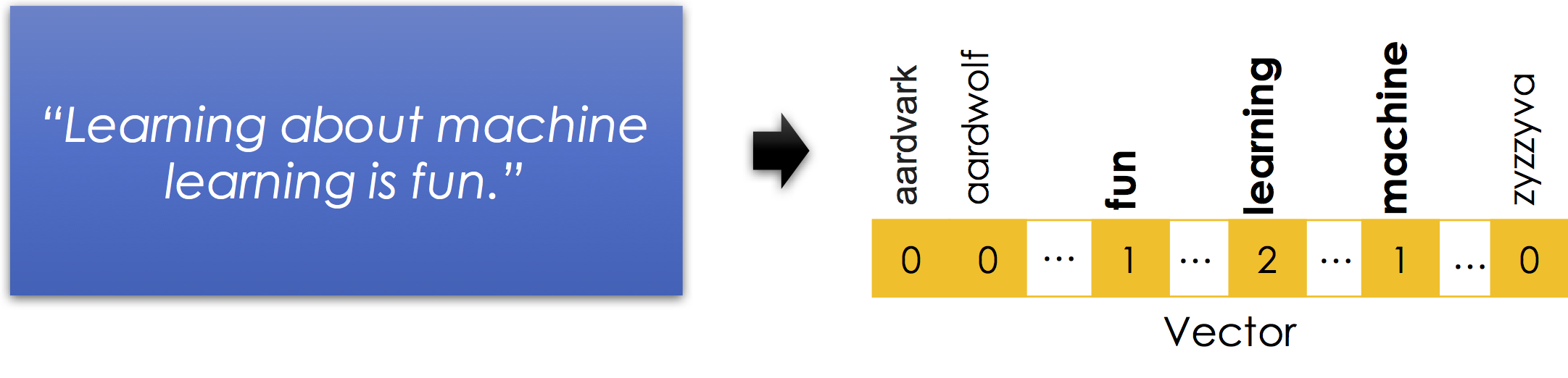Notice

1. Stop words are removed. Stop-words are words like is and about that in isolation contain very little information about the meaning of the sentence. Here is a good list of stop-words in many languages.
2. Word order information is lost. Nonetheless the vector still suggests that the sentence is about fun, machines, and learning. Thought there are many possible meanings learning machines have fun learning or learning about machines is fun learning ...
3. Capitalization and punctuation are typically removed.
4. Sparse Encoding: is necessary to represent the bag-of-words efficiently. There are millions of possible words (including terminology, names, and misspellings) and so instantiating a 0 for every word that is not in each record would be incredibly inefficient.

Why is it called a bag-of-words? A bag is another term for a multiset: an unordered collection which may contain multiple instances of each element.

# Break?¶

When professor Gonzalez was a graduate student at Carnegie Mellon University, he and several other computer scientists created the following art piece on display at the Gates Center:Notice

1. The unordered collection of words in the bag.
2. The stop words on the floor.
3. The missing broom. The original sculpture had a broom attached but the janitor got confused ....

## The N-Gram Encoding¶

The N-Gram encoding is a generalization of the bag-of-words encoding designed to capture limited ordering information. Consider the following passage of text:

The book was not well written but I did enjoy it.

If we re-arrange the words we can also write:

The book was well written but I did not enjoy it.

Moreover, local word order can be important when making decisions about text. The n-gram encoding captures local word order by defining counts over sliding windows. In the following example a bi-gram ($n=2$) encoding is constructed: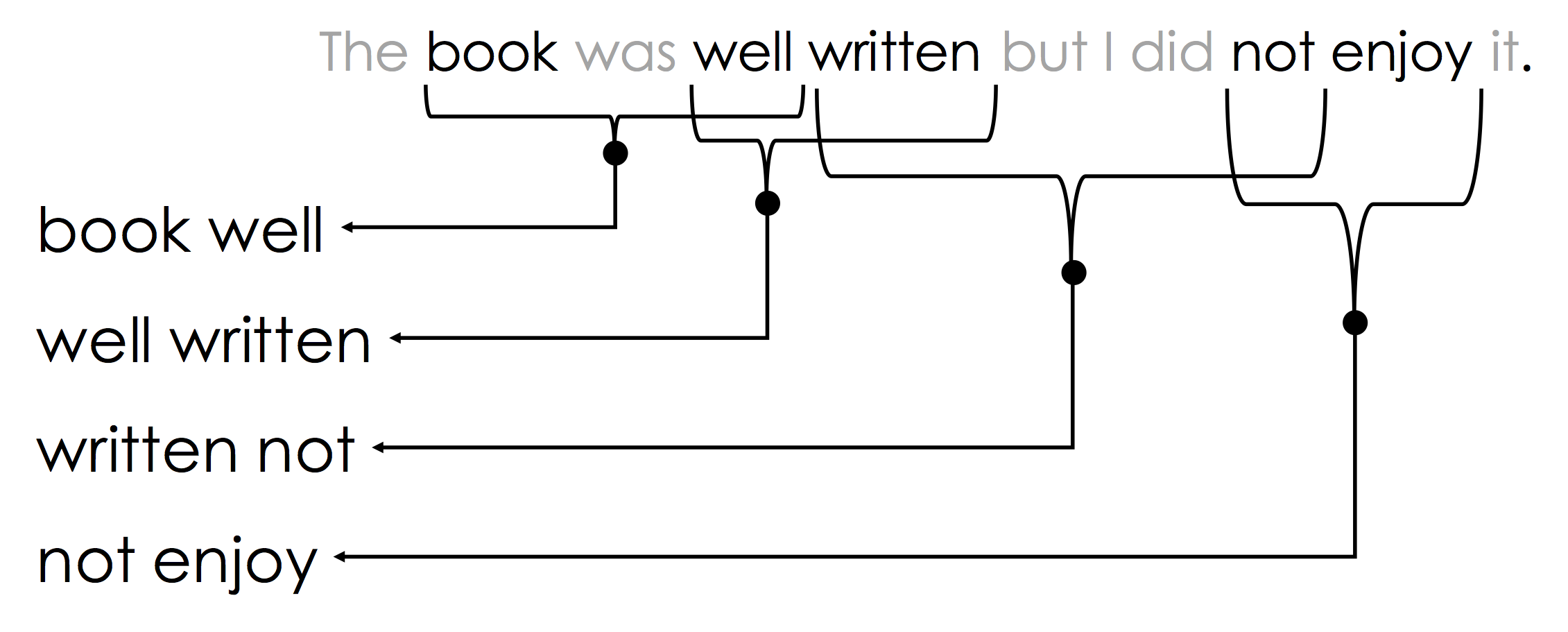The above n-gram would be encoded in the sparse vector: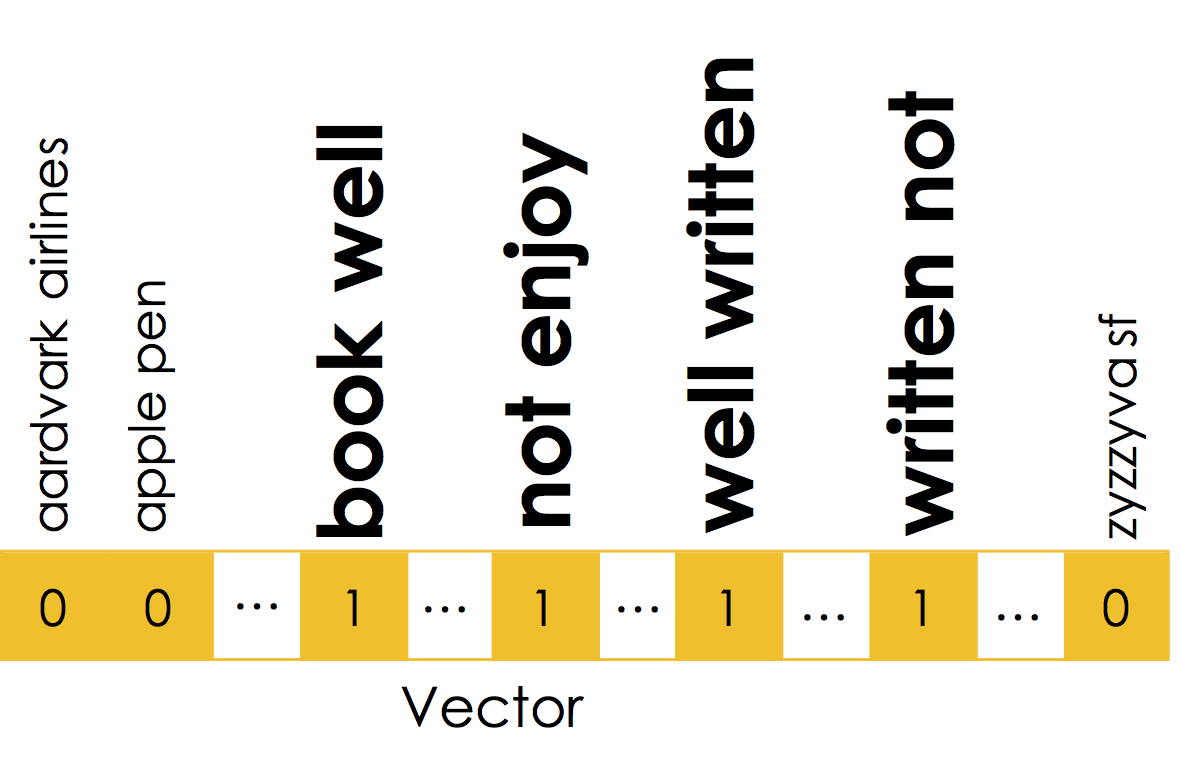Notice that the n-gram captures key pieces of sentiment information: "well written" and "not enjoy".

N-grams are often used for other types of sequence data beyond text. For example, n-grams can be used to encode genomic data, protein sequences, and click logs.

N-Gram Issues

1. The n-gram representation is hyper sparse and maintaining the dictionary of possible n-grams can be very costly. The hashing trick is a popular solution to approximate the sparse n-gram encoding. In the hashing trick each n-gram is mapped to a relatively large (e.g., 32bit) hash-id and the counts are associated with the hash index without saving the n-gram text in a dictionary. As a consequence, multiple n-grams are treated as the same.
2. As $N$ increase the chance of seeing the same n-grams at prediction time decreases rapidly.

## Implementing Bag-of-words and N-grams¶

In :
frost_text = [x for x in """
Some say the world will end in fire,
Some say in ice.
From what Ive tasted of desire
I hold with those who favor fire.
""".split("\n") if len(x) > 0]

frost_text

Out:
['Some say the world will end in fire,',
'Some say in ice.',
'From what Ive tasted of desire',
'I hold with those who favor fire.']
In :
from sklearn.feature_extraction.text import CountVectorizer

# Construct the tokenizer with English stop words
bow = CountVectorizer(stop_words="english")

# fit the model to the passage
bow.fit(frost_text)

Out:
CountVectorizer(analyzer='word', binary=False, decode_error='strict',
dtype=<class 'numpy.int64'>, encoding='utf-8', input='content',
lowercase=True, max_df=1.0, max_features=None, min_df=1,
ngram_range=(1, 1), preprocessor=None, stop_words='english',
strip_accents=None, token_pattern='(?u)\\b\\w\\w+\\b',
tokenizer=None, vocabulary=None)
In :
# Print the words that are kept
print("Words:",
list(zip(range(0,len(bow.get_feature_names())),bow.get_feature_names())))

Words: [(0, 'desire'), (1, 'end'), (2, 'favor'), (3, 'hold'), (4, 'ice'), (5, 'ive'), (6, 'say'), (7, 'tasted'), (8, 'world')]

In :
print("Sentence Encoding: \n")
# Print the encoding of each line
for (s, r) in zip(frost_text, bow.transform(frost_text)):
print(s)
print(r)
print("------------------")

Sentence Encoding:

Some say the world will end in fire,
(0, 1)	1
(0, 6)	1
(0, 8)	1
------------------
Some say in ice.
(0, 4)	1
(0, 6)	1
------------------
From what Ive tasted of desire
(0, 0)	1
(0, 5)	1
(0, 7)	1
------------------
I hold with those who favor fire.
(0, 2)	1
(0, 3)	1
------------------

In :
# Construct the tokenizer with English stop words
bigram = CountVectorizer(ngram_range=(1, 2))
# fit the model to the passage
bigram.fit(frost_text)

Out:
CountVectorizer(analyzer='word', binary=False, decode_error='strict',
dtype=<class 'numpy.int64'>, encoding='utf-8', input='content',
lowercase=True, max_df=1.0, max_features=None, min_df=1,
ngram_range=(1, 2), preprocessor=None, stop_words=None,
strip_accents=None, token_pattern='(?u)\\b\\w\\w+\\b',
tokenizer=None, vocabulary=None)
In :
# Print the words that are kept
print("\nWords:",
list(zip(range(0,len(bigram.get_feature_names())), bigram.get_feature_names())))

Words: [(0, 'desire'), (1, 'end'), (2, 'end in'), (3, 'favor'), (4, 'favor fire'), (5, 'fire'), (6, 'from'), (7, 'from what'), (8, 'hold'), (9, 'hold with'), (10, 'ice'), (11, 'in'), (12, 'in fire'), (13, 'in ice'), (14, 'ive'), (15, 'ive tasted'), (16, 'of'), (17, 'of desire'), (18, 'say'), (19, 'say in'), (20, 'say the'), (21, 'some'), (22, 'some say'), (23, 'tasted'), (24, 'tasted of'), (25, 'the'), (26, 'the world'), (27, 'those'), (28, 'those who'), (29, 'what'), (30, 'what ive'), (31, 'who'), (32, 'who favor'), (33, 'will'), (34, 'will end'), (35, 'with'), (36, 'with those'), (37, 'world'), (38, 'world will')]

In :
print("\nSentence Encoding: \n")
# Print the encoding of each line
for (s, r) in zip(frost_text, bigram.transform(frost_text)):
print(s)
print(r)
print("------------------")

Sentence Encoding:

Some say the world will end in fire,
(0, 1)	1
(0, 2)	1
(0, 5)	1
(0, 11)	1
(0, 12)	1
(0, 18)	1
(0, 20)	1
(0, 21)	1
(0, 22)	1
(0, 25)	1
(0, 26)	1
(0, 33)	1
(0, 34)	1
(0, 37)	1
(0, 38)	1
------------------
Some say in ice.
(0, 10)	1
(0, 11)	1
(0, 13)	1
(0, 18)	1
(0, 19)	1
(0, 21)	1
(0, 22)	1
------------------
From what Ive tasted of desire
(0, 0)	1
(0, 6)	1
(0, 7)	1
(0, 14)	1
(0, 15)	1
(0, 16)	1
(0, 17)	1
(0, 23)	1
(0, 24)	1
(0, 29)	1
(0, 30)	1
------------------
I hold with those who favor fire.
(0, 3)	1
(0, 4)	1
(0, 5)	1
(0, 8)	1
(0, 9)	1
(0, 27)	1
(0, 28)	1
(0, 31)	1
(0, 32)	1
(0, 35)	1
(0, 36)	1
------------------


## Bonus: Term Frequency Scaling¶

If we are encoding text in a particular domain (e.g., processing insurance claims) it is likely that there will be frequent terms (e.g., insurance or claim) that provide little information. However, because these terms occur frequently they can present challenges to some modeling techniques. In these cases, additional scaling may be applied to transform the bag-of-word or n-gram vectors to emphasize the more informative terms. One of the most common scalings techniques is the term frequency inverse document frequency (TF-IDF) which emphasizes words that are unique to a particular record. Because the notation is confusing, I have provided a pseudo code implementation. However, you should use a more efficient sparse implementation like those provided in scikit learn.

def tfidf(X):
"""
Input: X is a bag of words matrix (rows=records, cols=terms)
"""
(ndocs, nwords) = X.shape
tf = X / X.sum(axis=1)[:, np.newaxis]
idf = ndocs / (X > 0).sum(axis=0)
return tf * np.log(idf)


While these transformations are especially important when computing similarities between vector encodings of text. We will not cover these transformations in DS100 but it is worth knowing that they exist.

# Summary of Feature Encoding¶

Most machine learning (ML) and statistics techniques operate on multivariate real-valued domains (i.e., vectors). As a consequence, we need methods to encode non-continuous datatypes into meaningful continuous forms. We discussed:

1. one-hot (a.k.a. dummy variable) encoding transform categorical values into vectors of binary values with dimension equal to the number of possible values.
2. bag-of-words and n-gram encoding transform text into frequency statistics for individual terms and groups of terms.

We will now explore how feature transformations can be used to capture domain knowledge and encode complex relationships.